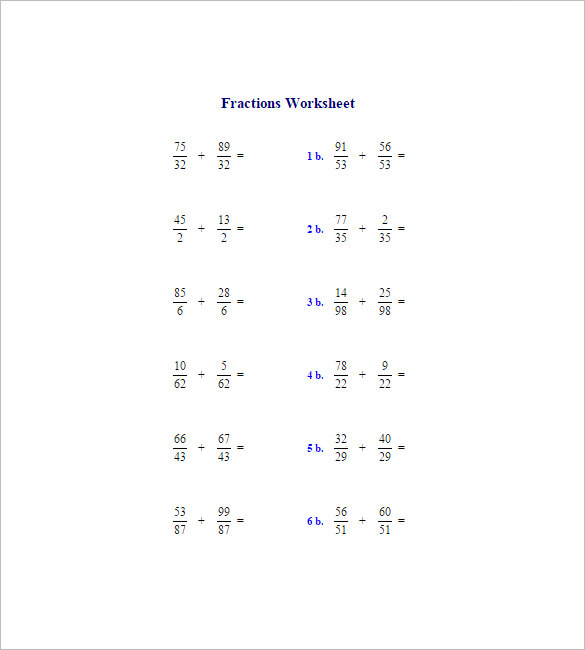Fraction addition and subtraction is quite a significant aspect of the secondary school syllabus. The secondary students should take care to practice these sums religiously to strengthen their maths know-how and a solid knowledge makes it easier to grasp complex arithmetic problems later on. Thus, the students are advised to practice with Adding and subtracting fractions worksheets. If you are a maths teacher, you are certainly required to provide your class with these worksheets.

## Add and Subtract Fractions Worksheet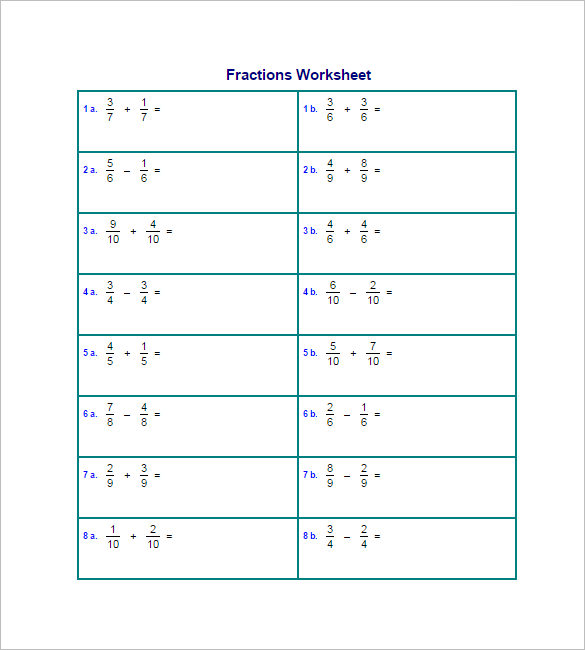## Adding and Subtracting Fractions Guide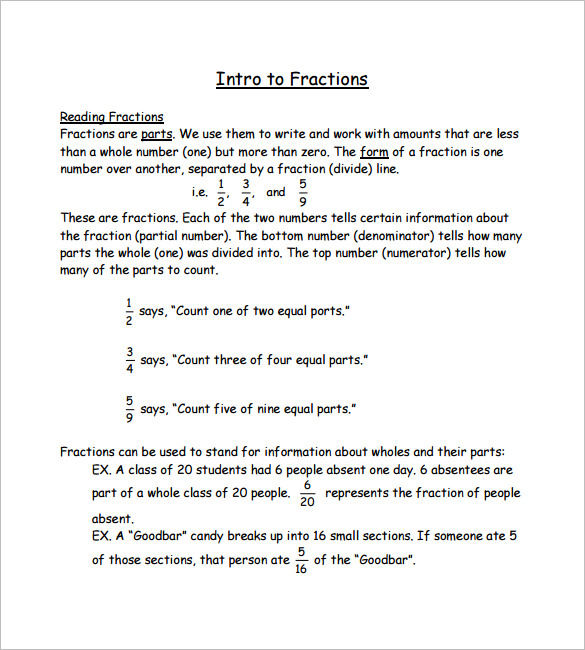## Adding and Subtracting Fractions Printable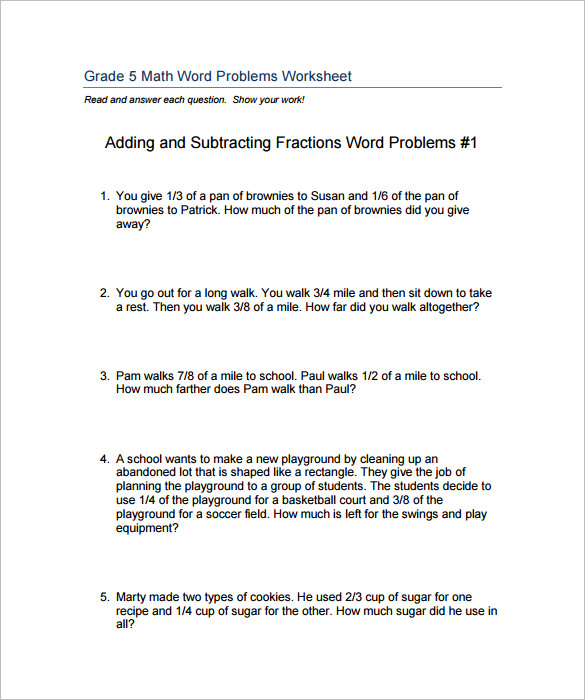## Sample Adding and Subtracting Fractions Word Problems Worksheets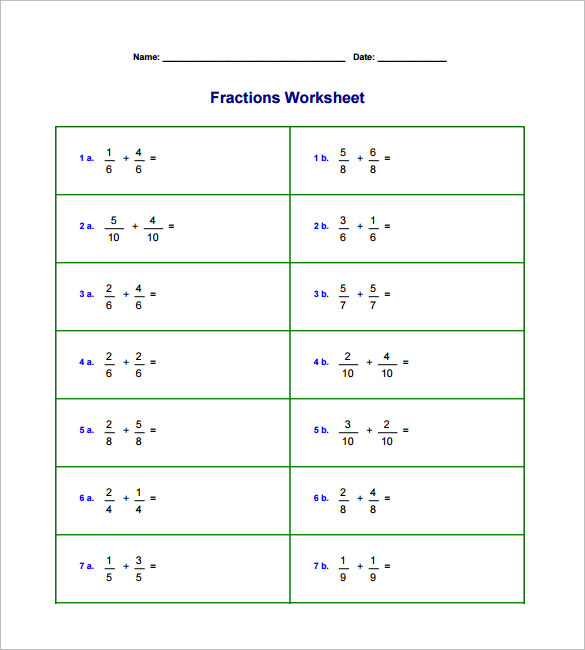## Adding and Subtracting Fractions Worksheets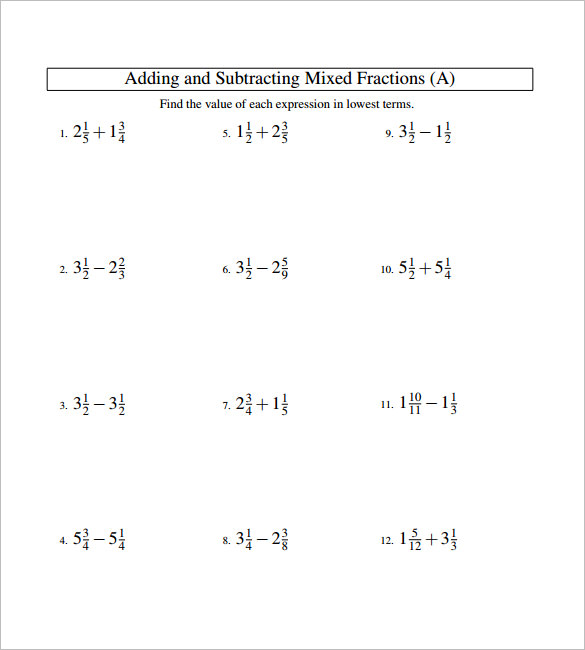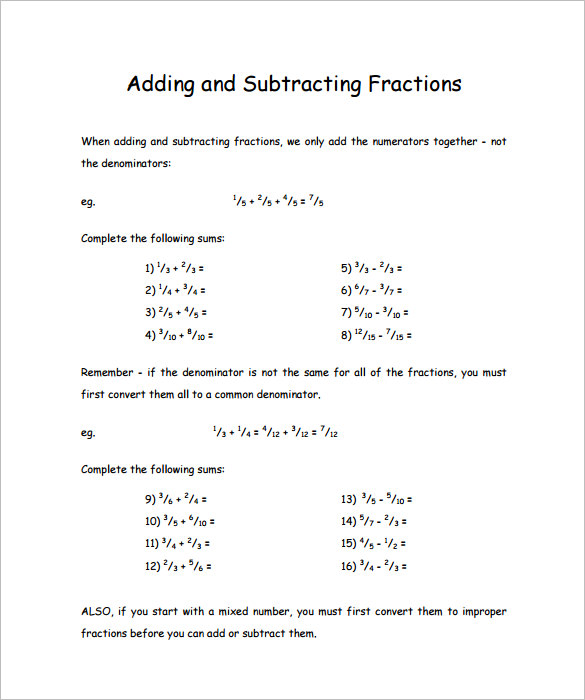## Adding and Subtracting Math Worksheet Examples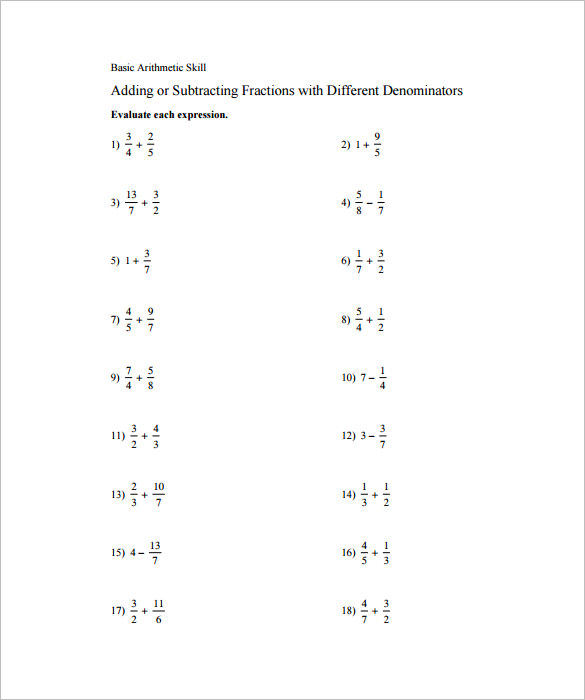## Adding Fractions Worksheet KS 3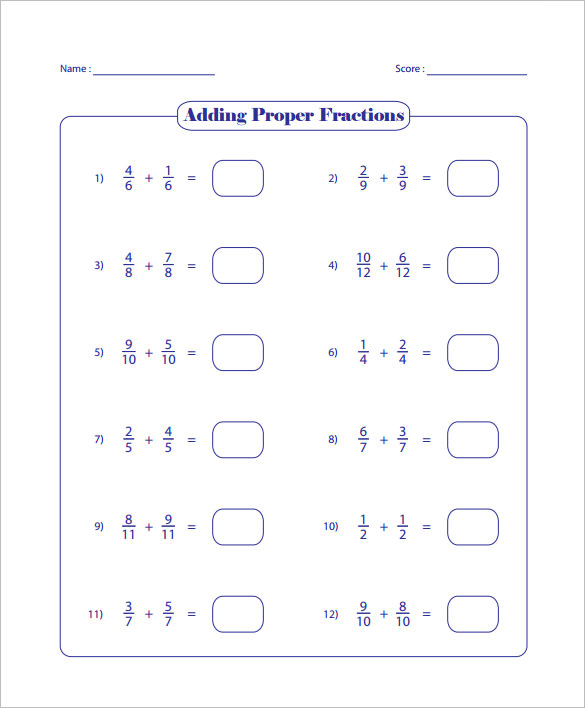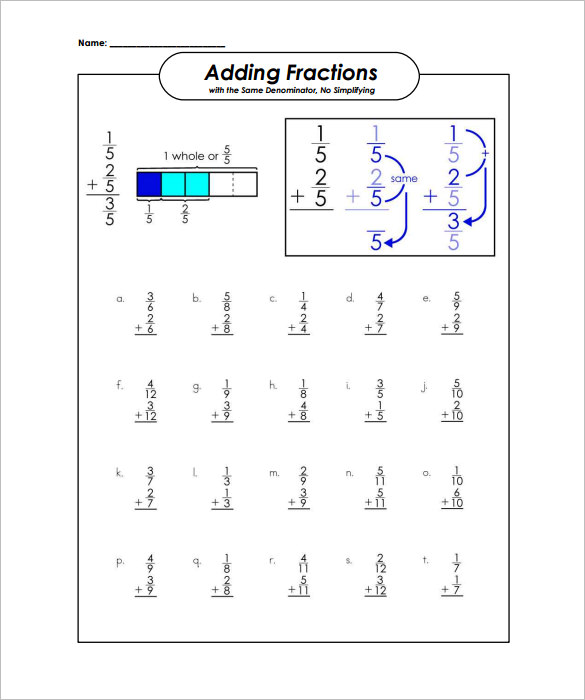## Addition of Fractions Worksheet Templates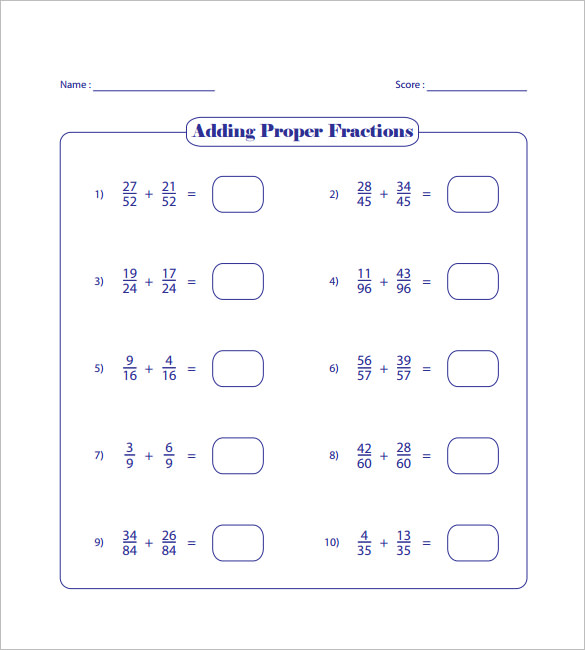## Subtracting Fractions Same Denominator Worksheets